#Streamline User Guide

### Site Tools

explain-inventory-dialog

# 6.9.4. Explain Inventory Calculation Dialog

Explain inventory calculation dialog is designed to show and explain Streamline’s planning process for each planning item. This dialog discovers the simulation modeling behind a purchase, replenishment, material requirements, and production plans generated by Streamline. It is especially useful to help understand the planning logic in the following complex cases as:

• two-echelon planning;
• material requirements planning; and
• combination of both.

First of all, we give a description of this dialog, and then show how it can be of help in particular situations using built-in examples.

## Description of the dialog

To open the Explain inventory calculation dialog:

1. Go to the Inventory planning tab.
2. Set the cursor at the planning item in question.
3. Press Ctrl + E, or right-click with the mouse and select the Explain calculation command from the context menu.

The Explain inventory calculation dialog appears (see figure below).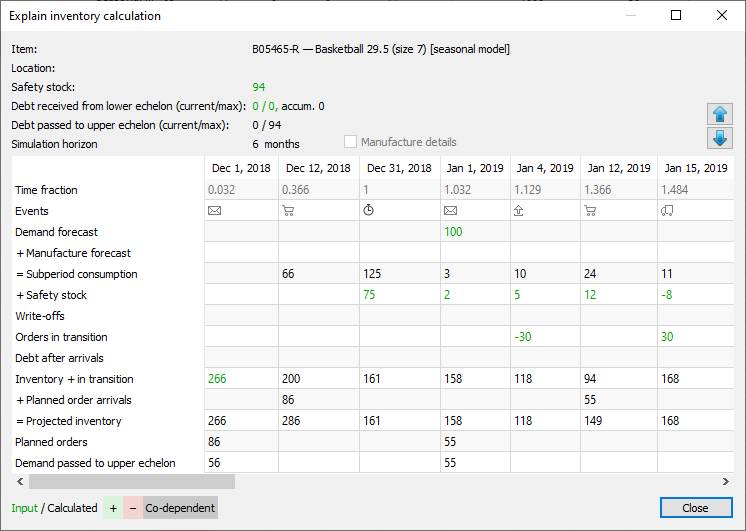This dialog is divided into two parts. The top part shows the properties of the currently selected planning item. The bottom part, a table displaying events that will happen to the item in the future based on the input data (actual events) and Streamline's simulation modeling (simulated events).

The dialog has its own color-coding helping you understand the math behind the Projected inventory levels.

### Planning Item Properites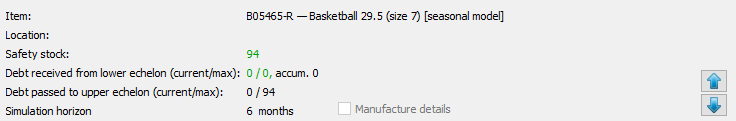• Item shows the code and the description of the item.
• Location shows the code and the description (if available) of the location.
• Debt received from lower echelon (current/max) shows the current, maximal, and accumulated safety stock debts. The current safety stock debt is received from the lower echelon. The maximal and the accumulated safety stock debts are calculated at the current echelon. These parameters are calculated for each planning item at each echelon in a multi-echelon model.
• Debt passed to upper echelon (current/max) displays the current and maximal safety stock debts that are calculated at the current echelon. The current safety stock is passsed to the upper echelon in the multi-echelon model.
• Current time shows the current day as a fraction of the data aggregation period (longer period). Streamline determines this date as the date of the last imported transaction. This date is also shown in the title of the Streamline application window.
• Simulation horizon shows the number of periods to simulate ahead. This number is specific for each planning item and is not less than the forecast horizon. To get all the necessary events calculated and shown within the Purchase plan and Projected inventory levels, Streamline extends the Forecast horizon to a certain number of periods. This number is specific for each planning item and depends on the Lead time and Order cycle set for the planning item.

### Table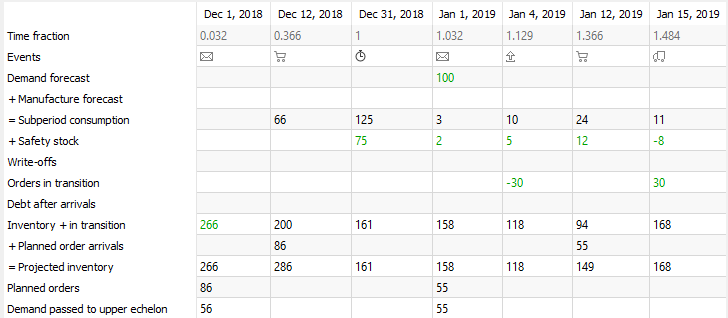• Each column header of the table displays the date the particular event happens.
• Time fraction is a numerical representation of the date shown in the table header. Zero time fraction corresponds to the last date of the previous data aggregation period. It is shown in the first column of the table.
• Events shows events that happen along the simulation. This column is the one that breaks data aggregation periods into subperiods. Date in the table header indicates the end of the subperiod. Each event has a unique icon. Icons and the corresponding events are shown in the table below.

Icon Event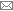Placement of a replenishment, purchase, or work order recommended by Streamline (simulation).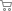Arrival of the order planned by Streamline (simulation).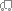Arrival of actual purchase or transfer order. You can view this order in the To receive tab of the In transition details dialog.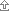Shipment of a sales order. You can view this order in the To ship tab of the In transition details dialog.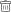Item write-off (simulation).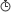End of the data aggregation period.
• Demand forecast indicates the final demand forecast for each data aggregation period. Streamline shows this value in the cell that corresponds to the first event of the period.
• Manufacture forecast shows the total consumption of the item (Material or Intermediate) got from the lower echelon. For example, assume we have a Material item which is used in two Finished items directly. In Streamline, relations between these items are represented by a two-echelon model, where the material is at the highest echelon and the finished products are at the lowest one. Consequently, the total demand for these two finished products is the manufacture forecast for the material.
• Subperiod consumption is the total of Demand forecast and Manufacture forecast brought to the subperiod time interval.
Streamline doesn't take into account the consumption for the past time period including the current date.
• Safety stock shows how the calculated safety stock is spread out along the first order cycle period. If safety stock is determined as the demand of the future periods, Streamline also shows safety stock for all the other order cycles by indicating the difference between the current and previous order cycle.
• Write-offs indicates how much inventory will be discarded due to the batches' shelf life within the simulation horizon. This row comes into play if inventory batches were imported, and shows the volumes of write-offs.
• Orders in transition shows quantities that will be shipped to customers (negative values) and received to locations (positive values) within the simulation horizon. This row pulls information from the To receive and To ship tabs of the In transition details dialog. These are actual (not simulated) events that are going to happen.
• Debt after arrival indicates the accumulated safety stock debt for each subperiod.
• Inventory + in transition. The main purpose of this row is to calculate and display the remaining on-hand level at the end of each order cycle period. Besides these goal quantities, the row displays how the on-hand level is decreased within each order cycle. These amounts are shown at the end of each subperiod. Streamline uses a color-coding to explain how this row is calculated (see figure below). This row shows the current item On hand in the first column of the table.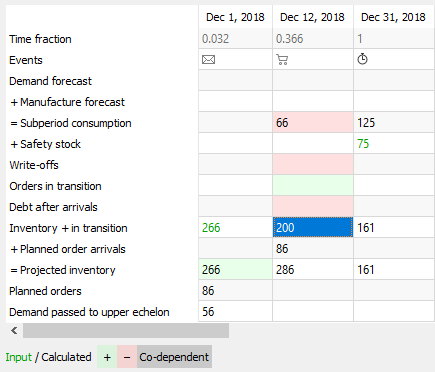• Planned order arrivals shows arrivals of orders planned by Streamline. Values of this row are tightly linked to the Planned orders row as they indicate the arrivals of the corresponding planned orders. Streamline displays this dependency using a color-coding once you set the cursor at the non-blank cell of the Planned orders row (see figure below).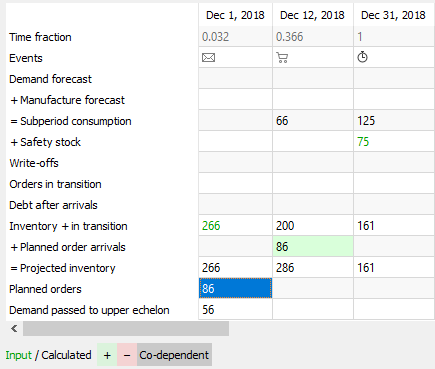• Projected inventory shows the projected inventory levels at the end of each subperiod. It is the sum of the Inventory + in transition and Planned orders arrivals (see figure below). Values of this row shown at the end of each data aggregation period make up the Projected inventory level report.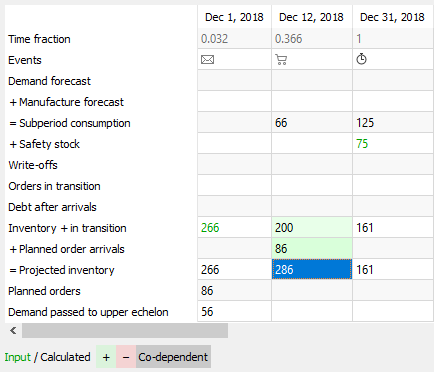• Planned orders shows Streamline's recommendations on how much and when to place a replenishment, purchase, or work order.
The first column of the table can be a little bit misleading, showing you an order placement (the email icon) on the past date. This is due to the zero time point which is used as a starting point to run the simulation from. Do not pay attention to the date in the header of this column. Streamline always calculates the Planned orders quantity for the first column on the current date.

### Buttons and Options

Buttons with Up and Down arrows on the right of the dialog allow navigating through the planning items.

Manufacture details option expands the table with all the items of the lower echelon which are linked to the current planning item based on the BOMs and shows the manufacture forecast for them.

### Color-coding

The color-coding legend for the table is shown in the figure below.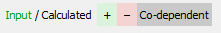• Green numbers are the inputs for the Planned orders and Projected inventory rows calculation.
• Black numbers are calculated values which, in turn, can also be considered as the inputs to the next level of calculations.

There are three color backgrounds for cells:

• Green and Red backgrounds indicate that the value is used with a positive and negative sign in the calculation accordingly.
• Grey background highlights cells which are co-dependent on each other.

## Examples

The primary purpose of the Explain inventory calculation dialog is to explain how the Purchase plan and the Projected inventory levels are calculated. In this section, we will show how to use this dialog to get these plans for the following built-in examples:

### Inventory Planning by Month

To show the capabilities of the dialog, we have slightly changed the input data of the project:

• added a customer order that is to be shipped on Jan 4 of 2019;
• changed the default Lead time to 10 days for item B05465-R (see figure below); and
• set Streamline to take safety stock as a demand of the future month.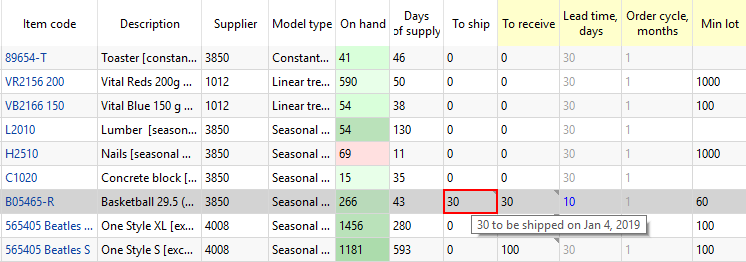The last modification allows Explain inventory calculation dialog to show safety stocks for each order cycle.

#### Current order quantity

Now, let's figure out, how the first planned order of 96 units is calculated - see the first cell of the Planned orders row in the figure below.

To determine the quantity to order currently (current date is Dec 1, 2018 in the example), Streamline uses the same formula as shown in the Understanding purchase plan and projected inventory levels article:

Current order = MAX(CEILING(MAX(0, D(OC) + Safety stock + Qty_to_ship(LT+OC) - Remaining), Rounding), Min lot), (1)

Remaining = MAX(0, MAX(0, On hand) + Qty_to_receive(LT + OC) - D(LT)).

Where:

• D(OC) is the order cycle demand starting from the lead time. D(OC) = 125 + 3 + 10 + 24 = 162.

As Lead time = 10 days that ends up on Dec 12, 2018 and the Order cycle is 1 month, we take the Subperiod consumption demand starting from the end of Dec 12, 2018 to the end of Jan 12, 2019. Since the dialog shows the dates the particular subperiod ends, we take the sum for the four subperiods staring from Dec 31, 2018.

• D(LT) is the demand forecast for the Lead time peirod.

D(LT) = Demand_forecast_for_period * Lead time, days / 30 = 197 * 10 / 30 = 66. This value is shown in the Subperiod consumption row on the date when the first Streamline's planned order arrives.

• Qty_to_ship(LT + OC) and Qty_to_receive(LT + OC) are the item quantities that are to be shipped/received within the Lead time plus Order cycle period.

As we have a shipment of 30 units that hit this interval (see figure above), Qty to ship = 30.

• Rounding = 12, Min lot = 60, On hand = 266.

Now, let's replace the parameters with the values:

Current order = MAX(CEILING(MAX(0, 162 + 94 + 30 - 200), 12), 60) = 96,

Remaining = MAX(0, MAX(0, 266) + 0 - 66) = 200.

We highlighted the values that are used in the calculation of the Current order quantity with positive and negative signs via green and red borders correspondingly in the figure above.

#### Projected inventory levels

To find out how the future on-hand levels at the end of each period are calculated, we will use two rows of the dialog table, Inventory + in transition and Planned order arrivals.

If you set the cursor at any cell of the Inventory + in transition row, you'll see the cells taking part in the calculation of the value. This row calculates the remaining on-hand at the end of each subperiod based on the:

• on-hand level at the end of the previous subperiod; and
• item consumption, possible write-off, safety stock debt, and in transition quantity in the currently selected subperiod (see figure below).In other words, this row shows the on-hand remaining at the end of each subperiod, however, except for the subperiods when Streamline's planned order arrivals happen. For those subperiods, it specifies the on-hand remaining not taking into account the arrival amount. These arrivals are shown in the Planned order arrivals row. The resulting projected inventory levels, which is the sum of the Inventory + in transition and Planned order arrivals rows, are indicated in the Projected inventory row. Consequently, the amounts at the end of each data aggregation period in this row make up the Projected inventory levels report (see figure below).

#### Purchase plan

In the first section of this example, we showed how Streamline calculates the Current order quantity. In this section, we explain how all the other order recommendations in the purchase plan are determined.

To calculate the subsequent order amounts, Streamline uses almost the same formula as formula (1):

Subsequent orderi = MAX(CEILING(MAX(0, `D(OCi)` + `Safety stocki` + `Qty_to_ship(OCi)` - `Qty_to_receive(OCi)` - `Remainingi-1`), Rounding), Min lot), `i = 2,…` (2)

where:

• `i` – the serial number of the order cycle period for which the quantity to order is calculated;
• `D(OCi)` – the demand during the `i`-th order cycle period;
• `Safety stocki` – the calculated safety stock for the `i`-th order cycle period;
• `Qty_to_ship(OCi)` – the anount of inventory that a to be shipped within the `i`-th order cycle period;
• `Qty_to_receive(OCi)` – the anount of inventory that a to be received within the `i`-th order cycle period;
• `Remainingi-1` – the remainig on-hand at the end of the previous (`i - 1`) order cycle period.

The values for all of these parameters can be directly found in the dialog table. The most interesting of them is the `Remainingi-1`, `i = 2,…`. As we said in the description of the dialog, the purpose of the Inventory + in transition is to calculate these remainings. The figure below highlights the values of the parameters that are used to get the amount for the second order.

We have intentionally set Streamline to determine safety stock as the demand for the given number of future periods (1 month in our example) to be able to show you how the order amount is calculated.

• `D(OC2)` = 11 + 52 + 3 + 27 = 93;
• `Safety stock2` = `Safety stock1` - 9 = 94 - 9 = 85;

Now, let's replace the parameters with the values:

Subsequent order2 = MAX(CEILING(MAX(0, 93 + 85 - 30 - 104), 12), 60) = MAX(CEILING(44, 12), 60) = 60.

This example is pretty simplified as it doesn't have imported:

To calculate the ordered quantities correctly in these more generic cases, the formulas (1) and (2) should account for the values from the mentioned rows.

explain-inventory-dialog.txt · Last modified: 2019/08/19 12:43 by admin

### Page Tools